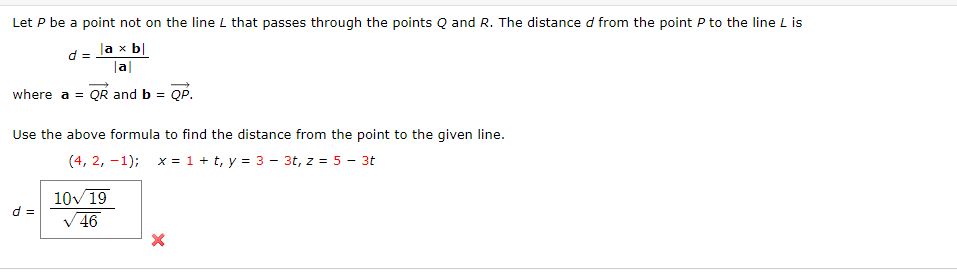Home / Answered Questions / Other / let-p-be-a-point-not-on-the-line-l-that-passes-through-the-points-q-and-r-the-distance-d-from-the-po-aw942

# (Solved): Let P Be A Point Not On The Line L That Passes Through The Points Q And R. The Distance D From The P...Let P be a point not on the line L that passes through the points Q and R. The distance d from the point P to the line L is de la xb| al where a = QR and b = OP. Use the above formula to find the distance from the point to the given line. (4, 2, -1); x = 1 + t, y = 3 - 3t, z = 5 - 30 10719 d = V 46

We have an Answer from Expert